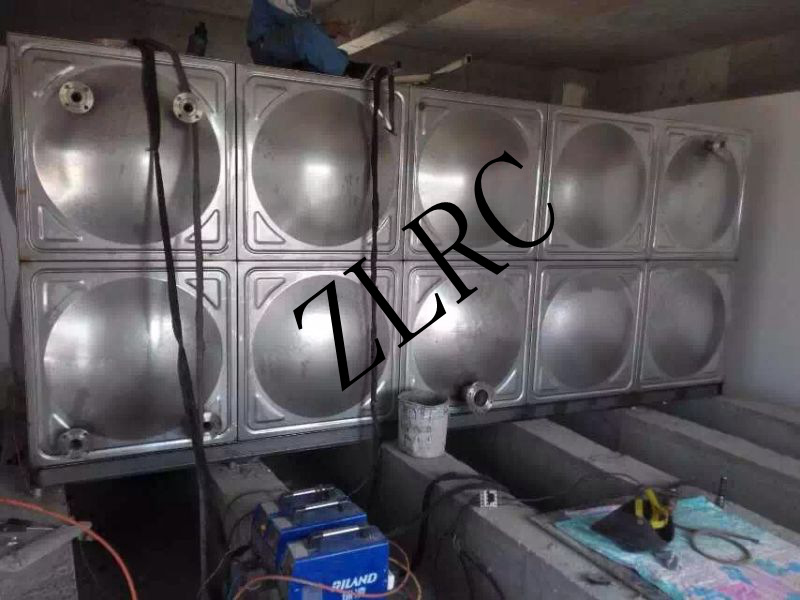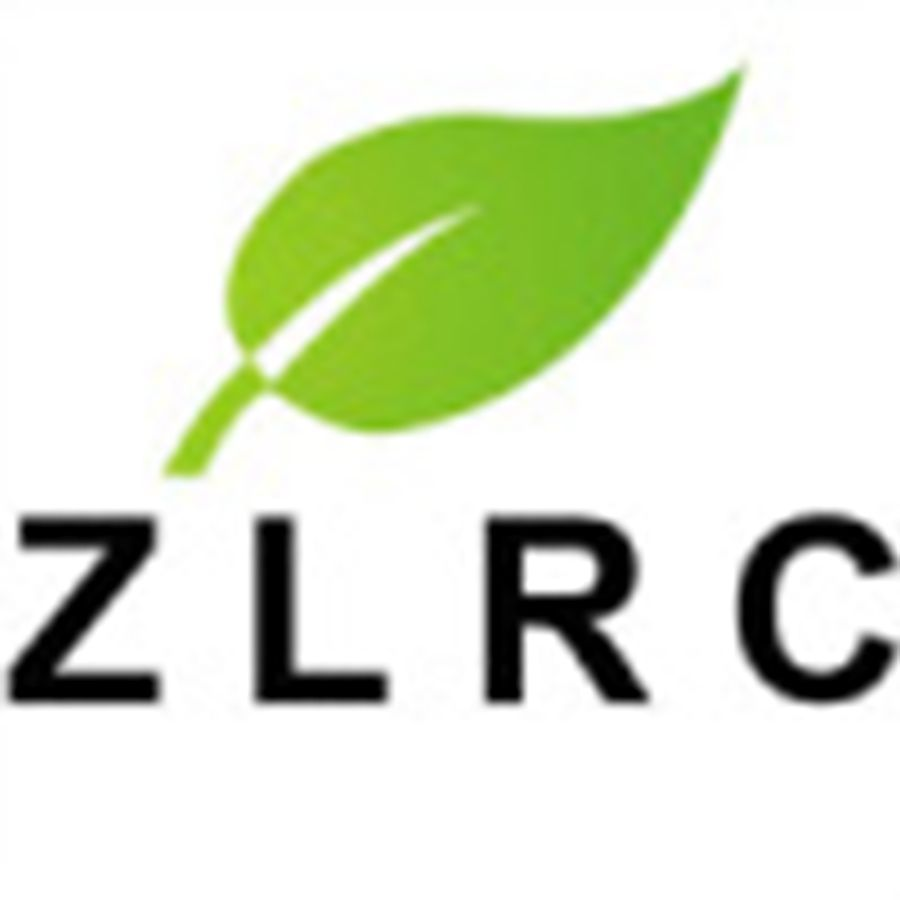## 北京正力润成环保设备有限公司# 北京正力润成生产安装各种规格 玻璃钢模压水箱 【不锈钢货源网buxiu.com】不锈钢水箱 质优价廉

## 诚信档案

### 企业名片

【友情提示】：来电请说明在304不锈钢价格,不锈钢钢丝绳,316l不锈钢板厂家-buxiu.com看到我们的，谢谢！

## 商品信息

≥1 立方

10000 立方

#### 有效期至：

r

r r

r

r
r

r

r 北京正力润成环保设备有限公司    水箱 r

r r  r r r r 产品介绍 r r水箱是工矿企业，民用住宅等公共供水系统不可缺少的组成部分，水箱分：SMC模压板组合式水箱、不锈钢板水箱、钢板搪瓷水箱及装备式不锈钢板水箱。
r1.SMC组合式水箱
r r

rr

r

rr

r

r  SMC组合式水箱是目前国际上普遍使用的新型水箱，具有水质好、无渗漏、重量轻、外型美观、使用寿命长和安装方便等优点。适用于工矿企事业单位、民用住宅、宾馆、饭店等公共建筑。作为生活用水、消防用水以及水质要求较高的食品、医药、卫生等行业必备的贮水设施。r

r r

r SMC模压单板是采用食品级树脂生产的SMC片材，经高温、高压、机械压制而成。在制造工艺上，采取机压成型的制作方法。增加了抗震、抗冲击的强度、克服了手糊制品强度不均匀的缺陷，提高了耐压强度和使用寿命；在外型设计上，板块中部呈凹陷弧度，提高了水箱的承压能力，同时板块四周设有45度和90度的凸缘，组装时不用边角连接件，更具有柔韧性和灵活性，水箱板块规格有：            1000×1000mm;1000×500mm;500×500mm。厚度有5mm、8mm、10mm、12mm、14mm、  16mm、18mm、20mm。其中5mm厚度板专用做水箱盖板。因而可任意拼装成所需容量的水箱。 r

r

r SMC模压单板系选用无毒树脂经过模压固化而成。
r 箱体浸泡水水质稳定，不含毒性，符合国家饮用水标准。
r 水箱密封采用专门研制的密封带。该密封带无毒、耐水、弹性大、永久变形小，使用期可在三十年以上。 r

r

r r

r

r 2. 不锈钢板水箱 r

r

rr

r3. 钢板搪瓷组合水箱 r r rr
r
r

r
• r

rr
rr
rr

r

r 正力润成环保设备有限公司的水箱优点 r

r

r 正力润成环保设备有限公司是一家专业从事水箱设计、生产、安装于一体的的綜合性企业，质量保证。 r

r

r
r

r

r 1.给水箱焊缝，材料好 r

r

r
r

r

r 装配式不锈钢水箱材质采用sus304δ2-δ4不锈钢板冲压出压强筋，并用不锈钢焊条整体焊接而成，结构简单，强度高。因箱体采用不锈钢板整体焊接，彻底折断了太阳光的
r
r照射，所以不会滋生藻类，使水质始终保持清洁。
r

r

r
r

r

r 针对不同设备，不同的运行状态，不同腐蚀渗漏形式，采用相应的技术产品，可快速、简单、有效保护设备，特别由于高分子复合材料所具有的抗化学腐蚀性能，粘结性能等综合性能，避免了传统补焊修复和传统耐腐材料保护所带来的不利影响，使修复后的设备寿命甚至超过新设备，从根本上解决腐蚀渗漏原因，帮助企业提高设备管理水平，降低维修维护成本，提高企业竞争力。 r

r

r
r

r

r 2.水箱规格： r

r

r
r

r

r O.5T-200T都可製作，并可依据客户要求承接方形、卧式及其它类形的特殊製作。 r

r

r
r

r

r 3.满水实验 r

r

r
r

r

r 水箱製作完毕后，将水箱完全充满水，经2~3小时后，用重0.5~1.5千克的锤铅沿焊缝两侧约150毫米的地方轻敲，不漏水为合格。若发现有漏水的地方，须重新焊接，再进行实验。 r

r

r
r

r

r 4.不锈钢水箱安装调试 r

r

r
r

r

r 不锈钢水箱基础可採用混凝土条形梁，或工字钢。不锈钢水箱顶部对角设有透气空气过滤装置，规格和数量根据进口尺寸大小由生产厂家确定装置。不锈钢水箱内部均使用拉筋。各管口法兰均为1.0MPa标准法兰。设计人员在佈置水箱位置时，应参照GB17051-1997二次供水设施卫生规范。水箱规格技术参数或方位与实际使用不适合时，由不锈钢水箱设计人员按实际需要提出确定。 r

r

r
r

r

r 5.售后服务-水箱施工 r

r

r
r

r

r 由专业的工程技术人员现场氩钨板熔化焊焊接。水箱的四周应有不小于500mm的检修空间，水箱顶至屋顶应有不小于400mm的空间，并确保施工现场整洁干净，水箱保温可根据用户具体要求，整体覆合保温。 r

r

r
r

r

r 经有关权威部门检测,各项指标达到或超过国外同类产品技术准,其製品具有如下优点:密度高,可根据不同应用场合,密度在200~500千克/立方进行调节，压缩强度大,可达4~20MPa，导热系数0.052.具有低导热系数,低透湿系数,低吸水率。 r

r

r
r

r

r 具有耐水解稳定性,抗老化,耐酸碱等化学药品性能优良，不易燃烧,阻燃性满足国家安全要求密度要求；-165-180度管托密度在300-500之间。 r

r

r
r

rrrrrrrrrrrrrrrrrrrrrrrrrrrrrrrrrrrrrrrrrrrrrrrrrrrrrrrrrrr  r r 容积（平方米） r r r r L（mm） r r r r B(mm) r r r r H(mm) r r r r 顶板(mm) r r r r 底板(mm) r r r r 边距(mm) r r r r 间距(mm) r r r r 数量 r r r r 1 r r r r 1000 r r r r 1000 r r r r 1000 r r r r 1.2 r r r r 1.5 r r r r 200 r r r r 600 r r r r 2 r r r r 6 r r r r 2000 r r r r 2000 r r r r 1500 r r r r 1.2 r r r r 1.5 r r r r 200 r r r r 5400 r r r r 4 r r r r 10 r r r r 2500 r r r r 2000 r r r r 2000 r r r r 1.2 r r r r 2 r r r r 200 r r r r 530 r r r r 5 r r r r 15 r r r r 3000 r r r r 2000 r r r r 2500 r r r r 1.2 r r r r 2 r r r r 300 r r r r 600 r r r r 2 r r r r r
r

r
r

r
• r
r r r r 公司介绍 r r北京正力润成环保设备有限公司是中国大型玻璃钢设备的销售商，是从事玻璃管道设备，缠绕罐设备，拉挤设备，格栅设备业务.销售的产品主要有：玻璃钢管道，玻璃钢罐，玻璃钢电缆桥架、玻璃钢冷却塔、玻璃钢水箱、玻璃钢风机、玻璃钢净化塔、玻璃钢制品等、中央空调末端产品的销售、及服务于一体的现代化企业。公司以，以人为本，创新务实，诚信高效，追求卓越为理念，以客户满意为标准，愿以优质的产品、良好的服务，与各界朋友真诚合作，共谋发展。
r r r r r r r 联系方式 r r r r

rr

r

r 联系人：郭女士 r

r

r 联系电话：13146067483， r

r

r 18513968493
r

r

r

r 邮箱：2268008712@qq.com r

r

r 网址：www.zlrcfrp.com r

r

r 地址：北京丰台区城南嘉园益城园16号楼 r

r

r 10层1-1018.（城南大道1座1018室） r

r r r r r 买家须知 r r
r
• r

r 一、按订货合同配置的附件 r

r

r 进水管水流出口上的水力浮球阀或普通浮球阀。（需要垂直安装阀，进水管应开在水箱顶部），水箱侧面上安装液位与水泵联锁时，箱内安装的液位继电器或信号器等。水箱侧面上安装液位计或溢流信号管。用户要求增设的其它附件，均由用户自行配置。 r

r
• r
• r

r
r

r

r 二、水箱验收 r

r

r
r

r

r 关闭出水口、排污口、打开进水阀门、待水箱盛满水后，3小时无渗漏异常声响及变形为合格。 r

r

r
r

r

r 三、不锈钢水箱使用说明: r

r

r
r

r

r 1.不锈钢水箱作为储存生活用水的二次设备，只能装生活用水（消防用水），不能装盐酸、硫酸或酸性、硷性含量较大的液体。 r

r

r
r

r

r 2.水箱在使用过程中，不要将其他笨重设备放置在水箱顶部，不要用硬物随意敲打或图画水箱版面及管件，有问题要预先通知厂家技术维修人员，维修焊接人员，要求是专门从事氩弧焊接工作的专业人员，维修时要把水放掉。 r

r

r
r

r

r 3.维护人员每月定时观察水箱内、外部主件及配件使用情况，有问题及时向厂家反映。 r

r
• r
• r

r 4.水箱入孔盖不要随意打开，防止水质受二次污染，水箱每年定时清洗、消毒1-2次。 r

r

r r

r
• r
r r

r
r

r
0相关评论

## 相关栏目

### 按排行字母分类：

A B C D E F G H I J K L M N O P Q R S T U V W X Y Z